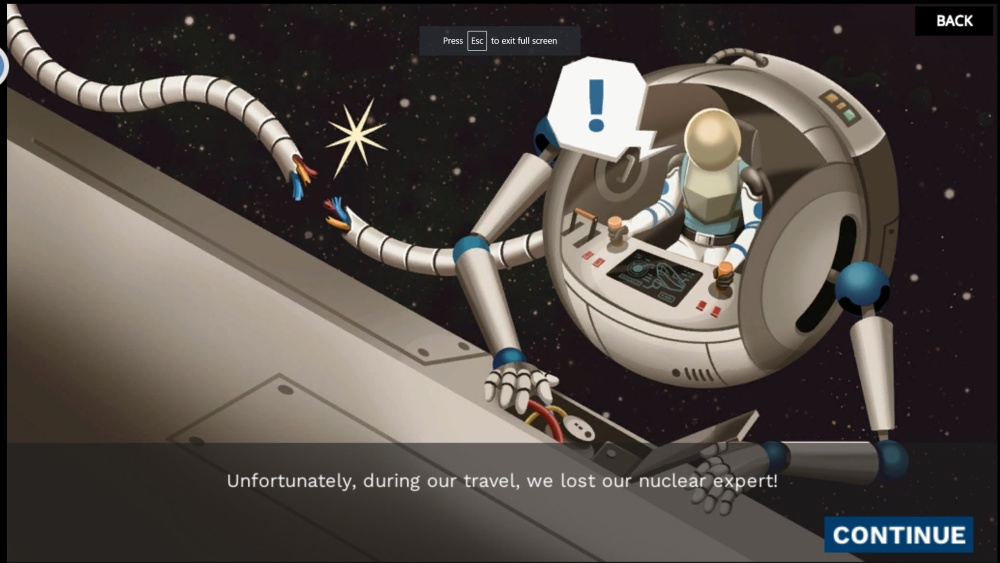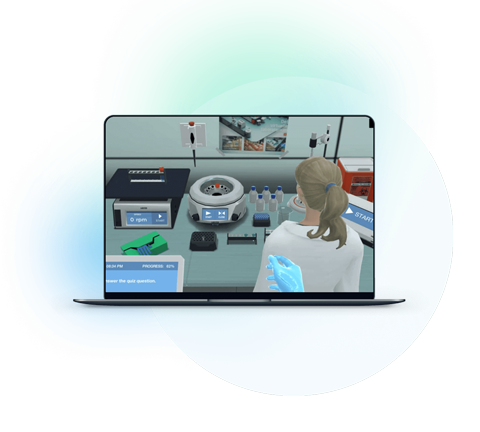Fusion is the process of combining two or more separate entities into a new whole. Nuclear fusion is a reaction in which two or more atomic nuclei combine to form one or more distinct atomic nuclei and subatomic particles (neutrons or protons).

Nuclear fission and fusion reactions are the two main types of nuclear reactions. Nuclear fusion is a reaction in which two or more light nuclei collide to form a heavier nucleus. The process of nuclear fusion occurs in elements with low atomic numbers, such as hydrogen. Nuclear fusion is the opposite of nuclear fission, in which heavy elements diffuse to form lighter elements. Both nuclear fusion and nuclear fission produce enormous amounts of energy.

Read on for some thoughts on why this can be a challenging topic for teachers and students, suggestions for remedying the situation, and thoughts on why virtual labs can make things easier.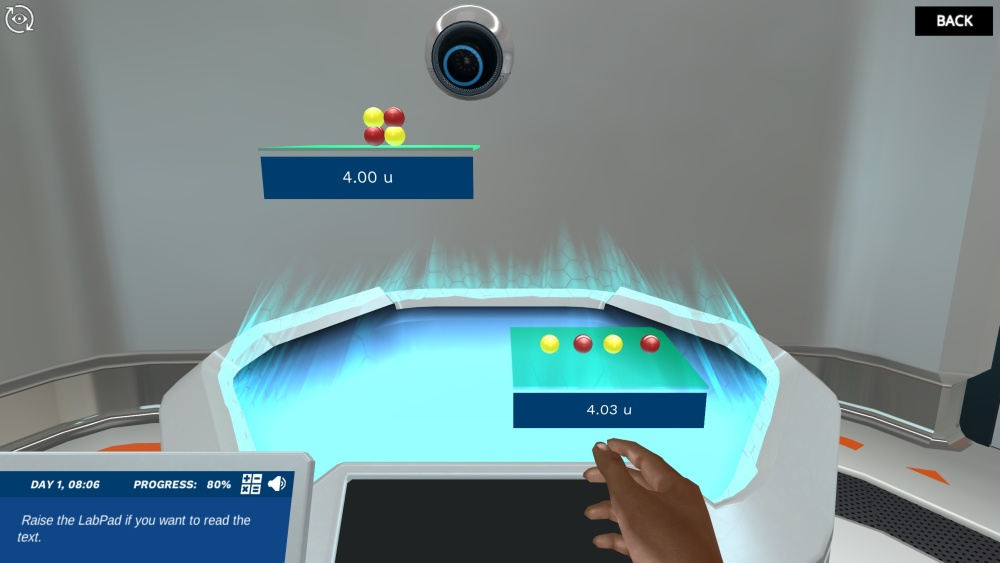Figure 1: A snippet from the nuclear fusion simulation Labster.

## Obstacles To Learning Nuclear Fusion

There are three reasons why nuclear fusion can be difficult for even the most active students.

### 1. Feels abstract

Because fusion occurs at the subatomic level, you can't see it. Not being able to visualize the process and not seeing its relevance to the real world can demoralize learning and make it difficult for students to stay motivated.

### 2. It’s content-heavy

Nuclide notation: The number of protons and neutrons in a nucleus is represented by nuclide notation. The mass number is the total number of nucleons (protons and neutrons). This is indicated in the superscript to the left of the item icon. The atomic number is the number of protons, it is indicated in the index to the left of the element symbol. When we subtract the atomic number from the mass number, the number of neutrons is gotten.

The element symbol indicates the element's name (e.g. He is helium). An element always has the same number of protons, so the atomic number is sometimes omitted. In this case, we can call the nuclide 32He 'helium three'.Figure 2: the annotated helium three nuclides.

### 3. It's complicated

Strength at its core: Two opposing forces compete in the nucleus of an atom: Protons have a positive electric charge, so they feel the electrostatic force. Each proton tries to repel the other. Both types of nucleons feel a strong attractive nuclear force within this distance. On this scale, the attraction due to the strong force is greater than the electrostatic repulsion between protons and this holds the nucleus together.  The strong force is within a very short range and the larger nuclei will not bind tightly. This results in an unstable nucleus that can decay or fission radioactively.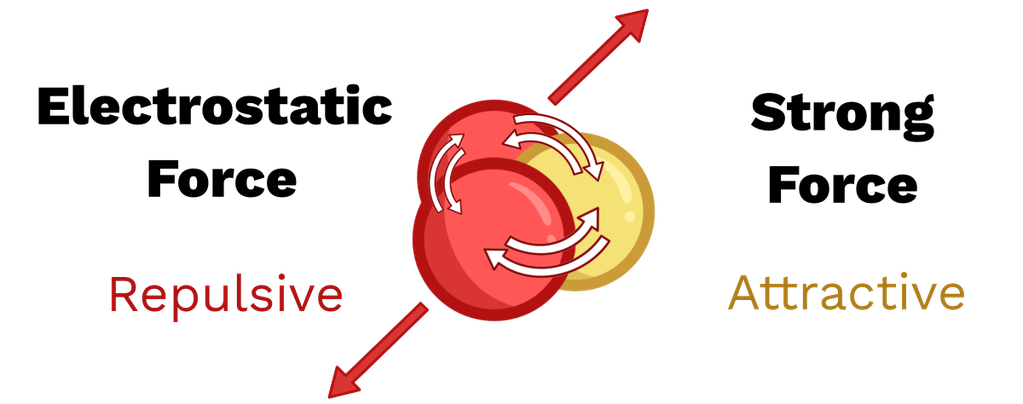Figure 3: A nucleus exhibits strong nuclear attraction (yellow) and electrostatic repulsion (red).

## Interesting Approach To Fusion

With these points in mind, here are five things you can add to nuclear fusion class to make it more engaging, accessible, and fun for you and your students.

### 1. Show the people behind the science

Nuclear fusion is the most dominant reaction in our observable universe, and it is this reaction that drives our sun and stars. However, the science and physics of nuclear fusion did not become clear until the 1920s, when British astrophysicist Arthur Eddington proposed that stars derive their energy from hydrogen fusion into helium. Eddington's theory was first published in 1926 in The Internal Structure of the Stars and laid the foundation for modern theoretical astrophysics.

Following Eddington's article, Robert d'Escort Atkinson and Fritz Hautermans gave the first calculations of the rate of nuclear fusion in stars. And at the same time, Ernest Rutherford was studying the structure of the atom. In his famous 1934 experiment, Rutherford demonstrated the fusion of deuterium into helium and found that a "major effect" was produced during the process. His student Mark Oliphant used an updated version of the apparatus, firing deuterium instead of hydrogen and discovering helium-3 and tritium, showing that heavy hydrogen nuclei can be tricked into reacting with each other. This is the first live demonstration of fusion in the laboratory. This understanding of nuclear fusion is linked to Hans Bethe's work on stellar nucleosynthesis, in which he describes how the sun and stars release energy through proton-proton chain reactions.

### 2. Relate it to the real world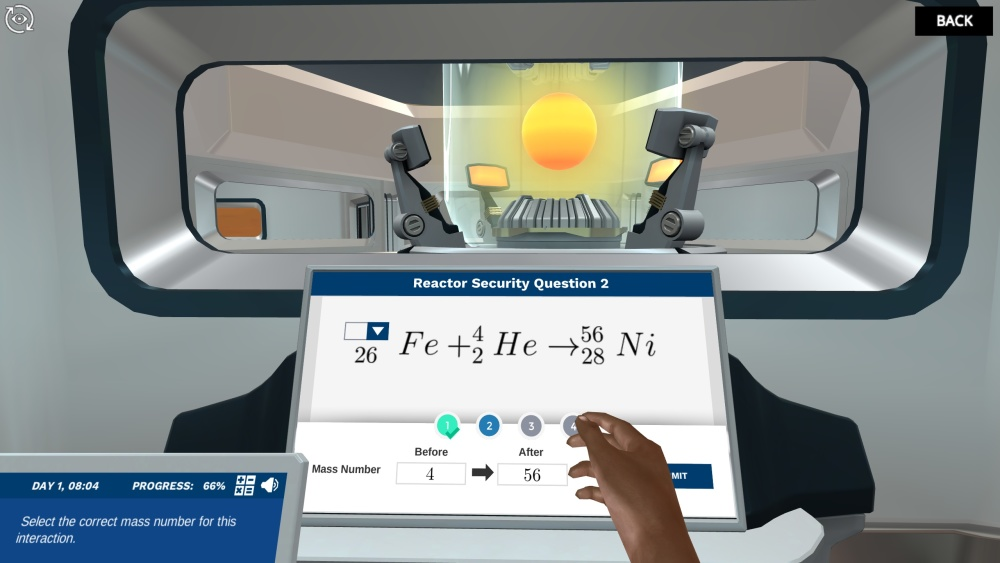Figure 4: A snippet from the nuclear fusion simulation Labster.

The Coulomb Barrier: The fusion of two atoms occurs when the nuclei approach a very small distance. Here the strong force pulls the nuclei together into a low-energy nucleus. The problem is, the nuclei also repel each other due to their electrostatic repulsion. Then, to combine, the particles must have sufficient kinetic energy to overcome the repulsion region (greater than 3 fm) and get to the region where the strong force is dominant. This energy "barrier" is known as the "Coulomb barrier," the energy required for two nuclei to be close enough to fuse. The energy required to trigger fusion is difficult to reproduce on Earth because it is so high. The only environments where this amount of energy can be found are the center of a star, its explosive supernova, and the big bang.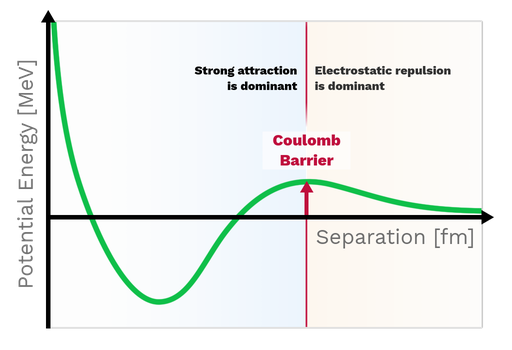Figure 5: A plot of the potential energy between two protons that changes with separation. The left side shows a region dominated by a strong power. The particles are at their lowest energy and fusion can occur. The right side shows the electrostatic area where the particles repel each other. The two are separated by a peak called the Coulomb barrier.

Einstein's equation

Science's most famous equation, E = mc2, tells us that mass "m" (measured in kg) can be converted into energy "E" (measured in J). The "exchange rate" between the two is the constant "c", the speed of light (3×108 m/s). Since this value is very large, we see that even a small amount of mass is converted into a large amount of energy; This is seen in nuclear fusion. Equation Energy (E) is equal to mass (m) times the speed of light (c) squared.Figure 7: Einstein's mass-energy equivalence equation.

Binding energy and mass defect: To break the nucleus, we must provide the energy to overcome the strong nuclear attraction of the nucleons. This amount of energy is called binding energy. Since energy is conserved, the nucleus must release the same amount of energy when formed from individual particles. This is where the energy released during nuclear fusion comes from.

We can observe this energy difference before and after fusion through the difference in mass; The nucleus is lighter than the total mass of its particles. When nucleons form a nucleus, some of their mass is converted into energy and released. The mass difference is called the mass defect and is related to the binding energy through E = mc2

### 3. Seeing is believing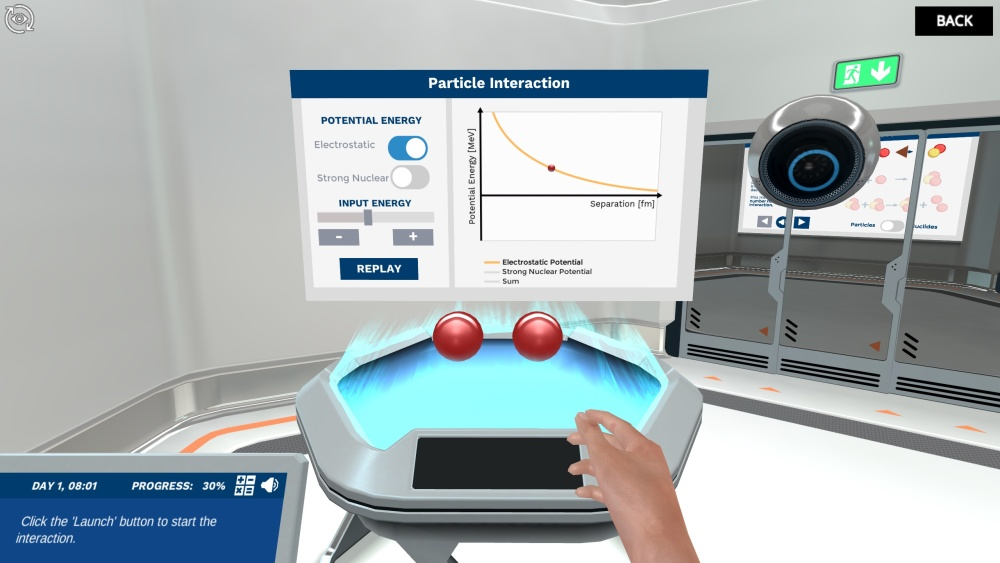Figure 7: A snippet from the nuclear fusion simulation Labster.

The law of conservation of nuclear physics

In physics, a quantity is conserved if it is the same before and after the interaction. The mass number is conserved in all interactions, including chemical reactions, radioactive decay, fusion, and fission.

The atomic number is only conserved in most cases, for example in chemical reactions and nuclear fission. However, it can be altered by weak interactions such as beta decay, which can occur with radioactivity and nuclear fusion where atomic number is not conserved. The Sun and other stars are powered by nuclear fusion. In a fusion reaction, two lighter nuclei join together to form a heavier nucleus. This process releases energy because the total mass of the resulting single nucleus is less than the mass of the original two nuclei. The remaining mass is converted into energy. Einstein's equation (E=mc2), which states that mass and energy can be converted into each other, explains why this process occurs. If scientists find a way to harness fusion energy in machines on Earth, it could become an important method for generating electricity.

### 4. Mnemonics can be useful

Visualization can be very helpful in understanding difficult topics like nuclear fusion, there are few options other than using mnemonics. The nuclide notation can be represented as MEAN which stands for

M - Mass number

E - Element symbol

A - Atomic number

N - number of Neutrons

### 5. Use a virtual laboratory simulation

A unique way to teach nuclear fusion, the future of energy, is through virtual lab simulations. At Labster, we are dedicated to providing fully interactive state-of-the-art lab simulations that use gamified elements such as storytelling and scoring systems in an immersive, 3D world.

Explore Labster's fusion simulations that allow students to learn about fusion through inquiry-based active learning. In the simulation, students embark on a mission to explore this state-of-the-art fusion reactor and learn all the science needed to put the power of the sun in the palm of their hand.